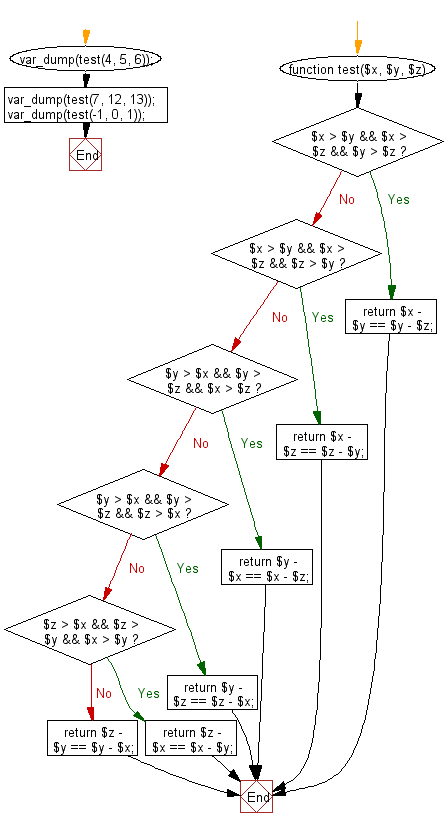﻿ Exercises: Check three given integers and return true if the difference between small and medium and the difference between medium and large is same - w3resource# PHP Exercises: Check three given integers and return true if the difference between small and medium and the difference between medium and large is same

## PHP Basic Algorithm: Exercise-58 with Solution

Write a PHP program to check three given integers (small, medium and large) and return true if the difference between small and medium and the difference between medium and large is same.

Sample Solution:

PHP Code :

``````<?php
function test(\$x, \$y, \$z)
{
if (\$x > \$y && \$x > \$z && \$y > \$z) return \$x - \$y == \$y - \$z;
if (\$x > \$y && \$x > \$z && \$z > \$y) return \$x - \$z == \$z - \$y;
if (\$y > \$x && \$y > \$z && \$x > \$z) return \$y - \$x == \$x - \$z;
if (\$y > \$x && \$y > \$z && \$z > \$x) return \$y - \$z == \$z - \$x;
if (\$z > \$x && \$z > \$y && \$x > \$y) return \$z - \$x == \$x - \$y;
return \$z - \$y == \$y - \$x;
}

var_dump(test(4, 5, 6));
var_dump(test(7, 12, 13));
var_dump(test(-1, 0, 1));
``````

Sample Output:

```bool(true)
bool(false)
bool(true)
```

Flowchart:PHP Code Editor:

What is the difficulty level of this exercise?

﻿

## PHP: Tips of the Day

\$_REQUEST: This SuperGlobal Variable is used to collect data submitted by a HTML Form

Example:

This code save in a php file and run in the browser.

```<!DOCTYPE html>
<html>
<body>

<form method="post" action="<?php echo \$_SERVER['PHP_SELF'];?>">
NAME: <input type="text" name="user">
<button type="submit">SUBMIT</button>
</form>
<?php
if (\$_SERVER["REQUEST_METHOD"] == "POST") {
\$name = htmlspecialchars(\$_REQUEST['user']);
if(empty(\$name)){
echo "Name is empty";
} else {
echo \$name;
}
}
?>
</body>
</html>
```

Output:

```Owen
```